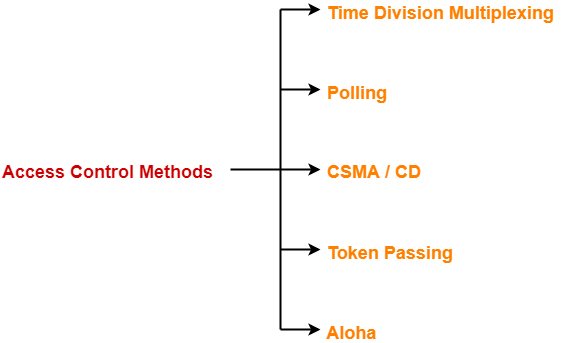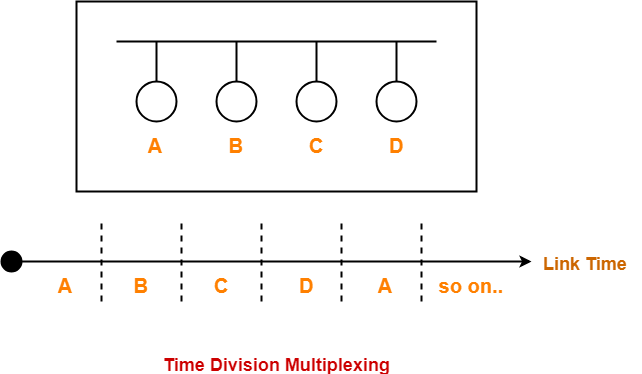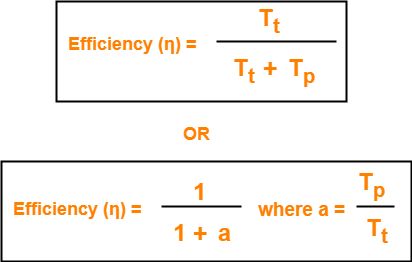## Access Control in Networking-

Before you go through this article, make sure that you have gone through the previous article on Access Control.

We have discussed-

• Access Control is a mechanism that controls the access of stations to the transmission link.
• There are various access control methods-## Time Division Multiplexing-

In Time Division Multiplexing (TDM),

• Time of the link is divided into fixed size intervals called as time slots or time slices.
• Time slots are allocated to the stations in Round Robin manner.
• Each station transmit its data during the time slot allocated to it.
• In case, station does not have any data to send, its time slot goes waste.

## Example-## Size Of Time Slots-

The size of each time slot is kept such that each station gets sufficient time for the following tasks-

• To put its data packet on to the transmission link
• Last bit of the packet is able to get out of the transmission link

Thus,

 Size of each time slot = Tt + Tp

where-

• Tt = Transmission delay
• Tp = Propagation delay

## NOTE-

To keep the size of time slots constant,

• We have assumed that all the stations want to send the packets of same size.
• This keeps Tt constant for all the stations.
• We have considered the worst case when both the stations are present at the two extreme ends.
• This ensures Tp will be maximum and all the stations will get sufficient time to propagate their data.

## Efficiency-

 Efficiency (η) = Useful Time / Total Time

• Useful time = Transmission delay of data packet = Tt
• Useless time = Propagation delay of data packet = Tp

Thus,## Important Formulas-

• Size of each time slot in Time Division Multiplexing = Tt + Tp
• Efficiency (η) = 1 / (1+a) where a = Tp / Tt
• Effective Bandwidth / Bandwidth Utilization / Throughput = Efficiency(η) x Bandwidth
• Maximum Available Effective Bandwidth = Total number of stations x Bandwidth requirement of 1 station

• If any station does not have the data to send during its time slot, then its time slot goes waste.
• This reduces the efficiency.
• This time slot could have been allotted to some other station willing to send data.

## Problem-

If transmission delay and propagation delay of a packet in Time Division Multiplexing is 1 msec each at 4 Mbps bandwidth, then-

1. Find the efficiency.
2. Find the effective bandwidth.
3. How many maximum stations can be connected to the network if each station requires 2 Kbps bandwidth?

## Solution-

Given-

• Transmission delay (Tt) = 1msec
• Propagation delay (Tp) = 1msec
• Bandwidth = 4 Mbps

## Part-01:

For a TDM Network,

 Efficiency (η) = 1 / 1+a where a = Tp / Tt

### Calculating Value Of ‘a’-

a = Tp / Tt

a = 1 msec / 1 msec

a = 1

Efficiency (η)

= 1 / (1+a)

= 1 / (1 + 1)

= 1 / 2

= 0.5

= 50%

## Part-02:

We know-

 Effective Bandwidth = Efficiency (η) x Bandwidth

Thus,

Effective Bandwidth

= 0.5 x 4 Mbps

= 2 Mbps

## Part-03:

We know-

 Maximum Effective Bandwidth= Total number of stations x Bandwidth requirement of 1 station

Let the total number of stations that can be connected be N.

Then, we have-

2 Mbps = N x 2 Kbps

N = 1000

Thus, maximum 1000 stations can be connected.

To gain better understanding about Time Division Multiplexing (TDM),

Watch this Video Lecture

Next Article-Polling | Access Control Method

Get more notes and other study material of Computer Networks.

Watch video lectures by visiting our YouTube channel LearnVidFun.